SBOS869 July   2017

PRODUCTION DATA.

1. Features
2. Applications
3. Description
4. Revision History
5. Pin Configuration and Functions
6. Specifications
7. Detailed Description
1. 7.1 Overview
2. 7.2 Functional Block Diagram
3. 7.3 Feature Description
4. 7.4 Device Functional Modes
8. Application and Implementation
1. 8.1 Application Information
2. 8.2 Typical Applications
1. 8.2.1 Low-side Current Measurement
2. 8.2.2 16-Bit Precision Multiplexed Data-Acquisition System
3. 8.2.3 Slew Rate Limit for Input Protection
9. Power-Supply Recommendations
10. 10Layout
11. 11Device and Documentation Support
1. 11.1 Device Support
1. 11.1.1 Development Support
2. 11.2 Documentation Support
5. 11.5 Community Resources
7. 11.7 Electrostatic Discharge Caution
8. 11.8 Glossary
12. 12Mechanical, Packaging, and Orderable Information

• D|8
• DGK|8
• D|8
• DGK|8

## 6 Specifications

### 6.1 Absolute Maximum Ratings

over operating free-air temperature range (unless otherwise noted)(1)
MIN MAX UNIT
Supply voltage, VS = (V+) – (V–) ±20
(+40, single supply)
V
Signal input pins Voltage Common-mode (V–) – 0.5 (V+) + 0.5 V
Differential (V+) – (V–) + 0.2
Current ±10 mA
Output short circuit(2) Continuous Continuous Continuous
Temperature Operating –40 150 °C
Junction 150
Storage, Tstg –65 150
Stresses beyond those listed under Absolute Maximum Ratings may cause permanent damage to the device. These are stress ratings only, which do not imply functional operation of the device at these or any other conditions beyond those indicated under Recommended Operating Conditions. Exposure to absolute-maximum-rated conditions for extended periods may affect device reliability.
Short-circuit to ground, one amplifier per package.

### 6.2 ESD Ratings

VALUE UNIT
V(ESD) Electrostatic discharge OPAx196 Human-body model (HBM), per ANSI/ESDA/JEDEC JS-001(1) ±3000 V
V(ESD) Electrostatic discharge OPA196 Charged-device model (CDM), per JEDEC specification JESD22-C101(2) ±1000 V
OPA2196 ±500 V
OPA4196 ±500 V
JEDEC document JEP155 states that 500-V HBM allows safe manufacturing with a standard ESD control process.
JEDEC document JEP157 states that 250-V CDM allows safe manufacturing with a standard ESD control process.

### 6.3 Recommended Operating Conditions

over operating free-air temperature range (unless otherwise noted)
MIN NOM MAX UNIT
Supply voltage, VS = (V+) – (V–) 4.5 (±2.25) 36 (±18) V
Specified temperature –40 125 °C

### 6.4 Thermal Information: OPA196

THERMAL METRIC(1) OPA196 UNIT
8 PINS 5 PINS
D (SOIC) DGK (VSSOP) DBV (SOT)
RθJA Junction-to-ambient thermal resistance 115.8 180.4 158.8 °C/W
RθJC(top) Junction-to-case(top) thermal resistance 60.1 67.9 60.7 °C/W
RθJB Junction-to-board thermal resistance 56.4 102.1 44.8 °C/W
ψJT Junction-to-top characterization parameter 12.8 10.4 1.6 °C/W
ψJB Junction-to-board characterization parameter 55.9 100.3 4.2 °C/W
RθJC(bot) Junction-to-case(bottom) thermal resistance N/A N/A N/A °C/W

### 6.5 Thermal Information: OPA2196

THERMAL METRIC(1) OPA2196 UNIT
8 PINS
D (SOIC) DGK (VSSOP)
RθJA Junction-to-ambient thermal resistance 107.9 158 °C/W
RθJC(top) Junction-to-case(top) thermal resistance 53.9 48.6 °C/W
RθJB Junction-to-board thermal resistance 48.9 78.7 °C/W
ψJT Junction-to-top characterization parameter 6.6 3.9 °C/W
ψJB Junction-to-board characterization parameter 48.3 77.3 °C/W
RθJC(bot) Junction-to-case(bottom) thermal resistance N/A N/A °C/W

### 6.6 Thermal Information: OPA4196

THERMAL METRIC(1) OPA4196 UNIT
14 PINS
D (SOIC) PW (TSSOP)
RθJA Junction-to-ambient thermal resistance 86.4 92.6 °C/W
RθJC(top) Junction-to-case(top) thermal resistance 46.3 27.5 °C/W
RθJB Junction-to-board thermal resistance 41.0 33.6 °C/W
ψJT Junction-to-top characterization parameter 11.3 1.9 °C/W
ψJB Junction-to-board characterization parameter 40.7 33.1 °C/W
RθJC(bot) Junction-to-case(bottom) thermal resistance N/A N/A °C/W

### 6.7 Electrical Characteristics: VS = ±4 V to ±18 V (VS = 8 V to 36 V)

at TA = 25°C, VCM = VOUT = VS / 2, and RL = 10 kΩ connected to VS / 2 (unless otherwise noted)
PARAMETER TEST CONDITIONS MIN TYP MAX UNIT
OFFSET VOLTAGE
VOS Input offset voltage VS = ±18 V ±25 ±100 µV
(V+) – 3.0 V < VCM < (V+) – 1.5 V See Common-Mode Voltage Range
VS = ±18 V,
VCM = (V+) – 1.5 V
±25 ±100
dVOS/dT Input offset voltage drift VS = ±18 V, VCM = (V+) – 3 V TA = –40°C to +125°C ±0.5 µV/°C
VS = ±18 V, VCM = (V+) – 1.5 V ±0.8
PSRR Power-supply rejection ratio TA = –40°C to +125°C ±0.3 ±1 µV/V
INPUT BIAS CURRENT
IB Input bias current ±5 ±20 pA
IOS Input offset current ±2 ±20 pA
NOISE
En Input voltage noise (V–) – 0.1 V < VCM < (V+) – 3 V f = 0.1 Hz to 10 Hz 1.4 µVPP
(V+) – 1.5 V < VCM < (V+) + 0.1 V f = 0.1 Hz to 10 Hz 7
en Input voltage noise density (V–) – 0.1 V < VCM < (V+) – 3 V f = 100 Hz 18 nV/√Hz
f = 1 kHz 15
(V+) – 1.5 V < VCM < (V+) + 0.1 V f = 100 Hz 53
f = 1 kHz 24
in Input current noise density f = 1 kHz 1.5 fA/√Hz
INPUT VOLTAGE
VCM Common-mode voltage range (V–) – 0.1 (V+) + 0.1 V
CMRR Common-mode rejection ratio VS = ±18 V,
(V–) – 0.1 V < VCM < (V+) – 3 V
120 140 dB
VS = ±18 V,
(V–) < VCM < (V+) – 3 V
TA = –40°C to +125°C 114 126
VS = ±18 V,
(V+) – 1.5 V < VCM < (V+)
96 120
TA = –40°C to +125°C 86 100
(V+) – 3 V < VCM < (V+) – 1.5 V See Typical Characteristics
INPUT IMPEDANCE
ZID Differential 100 || 1.6 MΩ || pF
ZIC Common-mode 1 || 6.4 1013Ω || pF
OPEN-LOOP GAIN
AOL Open-loop voltage gain VS = ±18 V,
(V–) + 0.6 V < VO < (V+) – 0.6 V,
RL = 2 kΩ
124 134 dB
TA = –40°C to +125°C 114 126
VS = ±18 V,
(V–) + 0.3 V < VO < (V+) – 0.3 V,
RL = 10 kΩ
126 140
TA = –40°C to +125°C 120 134
FREQUENCY RESPONSE
GBW Unity gain bandwidth 2.5 MHz
SR Slew rate VS = ±18 V, G = 1, 10-V step Rising 7.5 V/µs
Falling 5.5
ts Settling time To 0.01%, CL = 20 pF VS = ±18 V, G = 1, 2-V step 0.7 µs
VS = ±18 V, G = 1, 5-V step 1
To 0.001%, CL = 20 pF VS = ±18 V, G = 1, 2-V step 1.8
VS = ±18 V, G = 1, 5-V step 3.7
tOR Overload recovery time VIN × G = VS From overload to negative rail 0.4 µs
From overload to positive rail 1
THD+N Total harmonic distortion + noise G = 1, f = 1 kHz, VO = 3.5 VRMS 0.0012%
Crosstalk OPA2196 and OPA4196, at dc 150 dB
OPA2196 and OPA4196, f = 100 kHz 130 dB
OUTPUT
VO Voltage output swing from rail Positive rail No load 5 15 mV
RL = 10 kΩ 50 110
RL = 2 kΩ 200 500
Negative rail No load 5 15
RL = 10 kΩ 50 110
RL = 2 kΩ 200 500
ISC Short-circuit current VS = ±18 V ±65 mA
CL Capacitive load drive See Typical Characteristics
ZO Open-loop output impedance f = 1 MHz, IO = 0 A, See Figure 19 700 Ω
POWER SUPPLY
IQ Quiescent current per amplifier IO = 0 A 140 200 µA
TA = –40°C to +125°C 250
TEMPERATURE
Thermal protection 180 °C
Thermal hysteresis 30 °C

### 6.8 Electrical Characteristics: VS = ±2.25 V to ±4 V (VS = 4.5 V to 8 V)

at TA = +25°C, VCM = VOUT = VS / 2, and RL = 10 kΩ connected to VS / 2 (unless otherwise noted)
PARAMETER TEST CONDITIONS MIN TYP MAX UNIT
OFFSET VOLTAGE
VOS Input offset voltage VS = ±2.25V,
VCM = (V+) – 3 V
±25 ±100 µV
(V+) – 3.0 V < VCM < (V+) – 1.5 V See Common-Mode Voltage Range
VS = ±3V,
VCM = (V+) – 1.5 V
±25 ±100
dVOS/dT Input offset voltage drift VS = ±2.25V, VCM = (V+) – 3 V TA = –40°C to +125°C ±0.5 µV/°C
VS = ±2.25V, VCM = (V+) – 1.5 V ±0.5
PSRR Power-supply rejection ratio TA = –40°C to +125°C, VCM = VS / 2 – 0.75 V ±1 µV/V
INPUT BIAS CURRENT
IB Input bias current ±5 ±20 pA
IOS Input offset current ±2 ±20 pA
NOISE
En Input voltage noise (V–) – 0.1 V < VCM < (V+) – 3 V f = 0.1 Hz to 10 Hz 1.4 µVPP
(V+) – 1.5 V < VCM < (V+) + 0.1 V f = 0.1 Hz to 10 Hz 7
en Input voltage noise density (V–) – 0.1 V < VCM < (V+) – 3 V f = 100 Hz 18 nV/√Hz
f = 1 kHz 15
(V+) – 1.5 V < VCM < (V+) + 0.1 V f = 100 Hz 53
f = 1 kHz 24
in Input current noise density f = 1 kHz 1.5 fA/√Hz
INPUT VOLTAGE
VCM Common-mode voltage range (V–) – 0.1 (V+) + 0.1 V
CMRR Common-mode rejection ratio VS = ±2.25 V,
(V–) – 0.1 V < VCM < (V+) – 3 V
96 110 dB
VS = ±2.25 V,
(V–) < VCM < (V+) – 3 V
TA = –40°C to +125°C 90 104
VS = ±2.25 V,
(V+) – 1.5 V < VCM < (V+)
96 120
TA = –40°C to +125°C 84 100
(V+) – 3 V < VCM < (V+) – 1.5 V See Typical Characteristics
INPUT IMPEDANCE
ZID Differential 100 || 1.6 MΩ || pF
ZIC Common-mode 1 || 6.4 1013Ω || pF
OPEN-LOOP GAIN
AOL Open-loop voltage gain VS = ±2.25V,
(V–) + 0.6 V < VO < (V+) – 0.6 V,
RL = 2 kΩ
110 120 dB
TA = –40°C to +125°C 100 114
VS = ±2.25V,
(V–) + 0.3 V < VO < (V+) – 0.3 V,
RL = 10 kΩ
110 126
TA = –40°C to +125°C 106 120
FREQUENCY RESPONSE
GBW Unity gain bandwidth 2.2 MHz
SR Slew rate VS = ±2.25V, G = 1, 1-V step Rising 6.5 V/µs
Falling 5.5
tOR Overload recovery time VIN × G = VS From overload to negative rail 0.4 µs
From overload to positive rail 1
Crosstalk OPA2196 and OPA4196, at dc 150 dB
OPA2196 and OPA4196, f = 100 kHz 130 dB
OUTPUT
VO Voltage output swing from rail Positive rail No load 5 15 mV
RL = 10 kΩ 15 110
RL = 2 kΩ 60 500
Negative rail No load 5 15
RL = 10 kΩ 15 110
RL = 2 kΩ 60 500
ISC Short-circuit current VS = ±2.25V ±30 mA
CL Capacitive load drive See Typical Characteristics
ZO Open-loop output impedance f = 1 MHz, IO = 0 A, see Figure 19 700 Ω
POWER SUPPLY
IQ Quiescent current per amplifier IO = 0 A 140 200 µA
TA = –40°C to +125°C 250
TEMPERATURE
Thermal protection 180 °C
Thermal hysteresis 30 °C

### Table 1. Table of Graphs

DESCRIPTION FIGURE
Offset Voltage vs Common-Mode Voltage Figure 1
Open-Loop Gain and Phase vs Frequency Figure 2
Closed-Loop Gain and Phase vs Frequency Figure 3
Input Bias Current vs Common-Mode Voltage Figure 4
Input Bias Current vs Temperature Figure 5
Output Voltage Swing vs Output Current (maximum supply) Figure 6, Figure 7
CMRR and PSRR vs Frequency Figure 8
CMRR vs Temperature Figure 9
PSRR vs Temperature Figure 10
0.1-Hz to 10-Hz Noise Figure 11
Input Voltage Noise Spectral Density vs Frequency Figure 12
THD+N Ratio vs Frequency Figure 13
THD+N vs Output Amplitude Figure 14
Quiescent Current vs Supply Voltage Figure 15
Quiescent Current vs Temperature Figure 16
Open Loop Gain vs Temperature Figure 17, Figure 18
Open Loop Output Impedance vs Frequency Figure 19
Small Signal Overshoot vs Capacitive Load (100-mV output step) Figure 20, Figure 21
No Phase Reversal Figure 22
Small-Signal Step Response (100 mV) Figure 24, Figure 25
Large-Signal Step Response Figure 26, Figure 27
Settling Time Figure 28, Figure 29, Figure 30, Figure 31
Short-Circuit Current vs Temperature Figure 32
Maximum Output Voltage vs Frequency Figure 33
Propagation Delay Rising Edge Figure 34
Propagation Delay Falling Edge Figure 35
At TA = 25°C, VS = ±18 V, VCM = VS / 2, RL = 10 kΩ connected to VS / 2, and CL = 100 pF, unless otherwise noted.Figure 1. Offset Voltage vs Common-Mode Voltage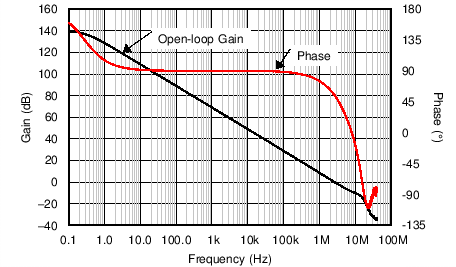Figure 2. Open-Loop Gain and Phase vs Frequency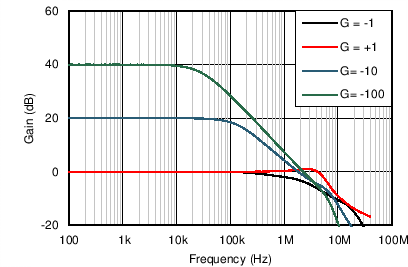Figure 3. Closed-Loop Gain vs Frequency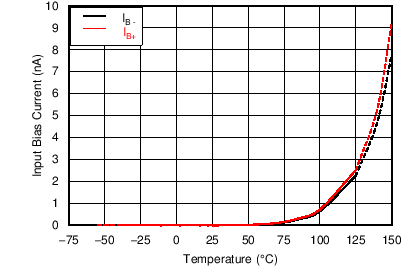Figure 5. Input Bias Current vs Temperature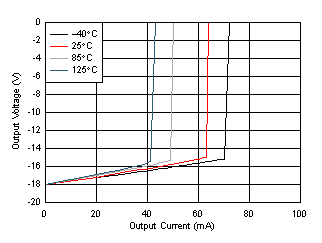Sinking
Figure 7. Output Voltage Swing vs Output Current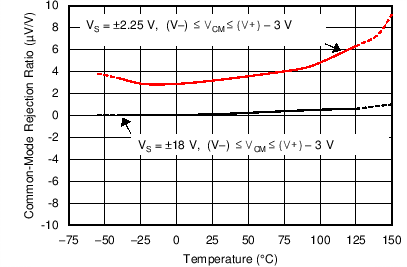Figure 9. CMRR vs Temperature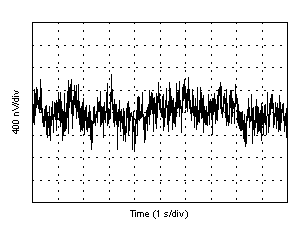Figure 11. 0.1-Hz to 10-Hz Noise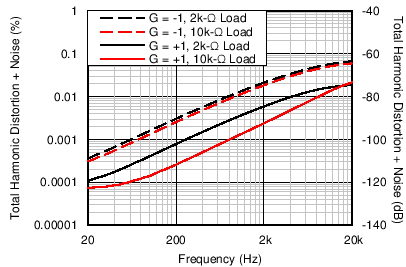Figure 13. THD+N vs Frequency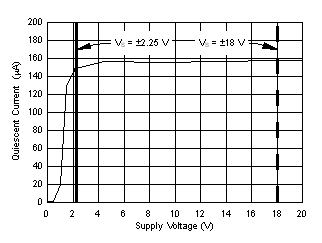Figure 15. Quiescent Current vs Supply Voltage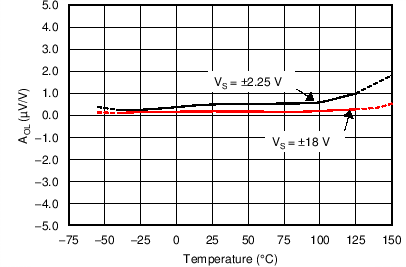RL = 10 kΩ
Figure 17. Open-Loop Gain vs TemperatureFigure 19. Open-Loop Output Impedance vs Frequency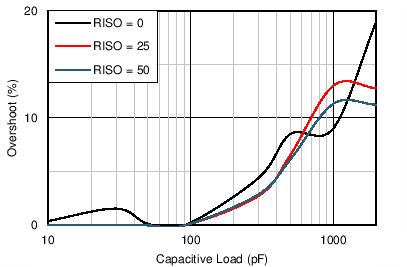G = 1, 100-mV output step
Figure 21. Small-Signal Overshoot vs Capacitive Load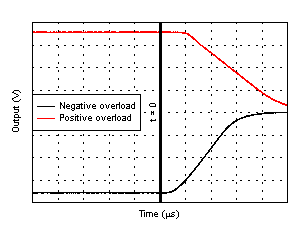VS = ±18 V, G = –10 V/V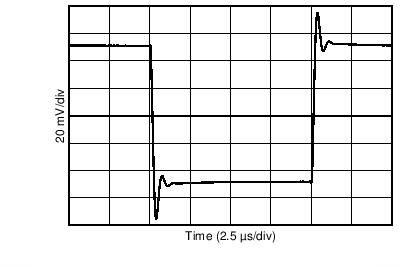G = –1, RL = 1 kΩ, CL = 10 pF
Figure 25. Small-Signal Step ResponseG = –1, RL = 1 kΩ, CL = 10 pF
Figure 27. Large-Signal Step Response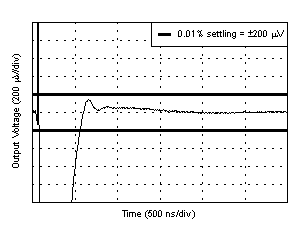Gain = 1, 2-V step, falling, step applied at t = 0 µs
Figure 29. 0.01% Settling Time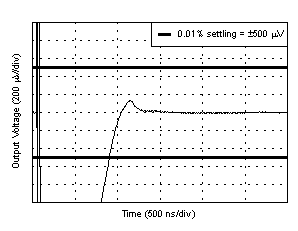Gain = 1, 5-V step, falling, step applied at t = 0 µs
Figure 31. 0.01% Settling Time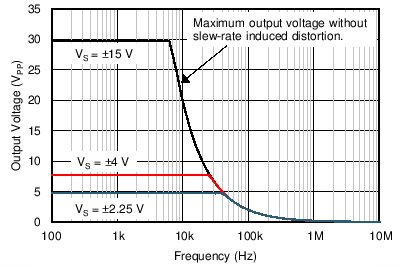Figure 33. Maximum Output Voltage vs Frequency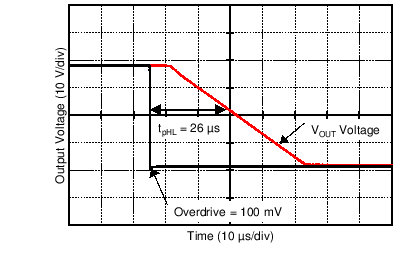Figure 35. Propagation Delay Falling Edge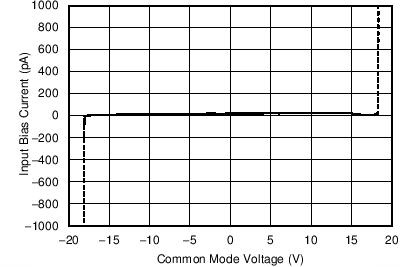Figure 4. Input Bias Current vs Common-Mode Voltage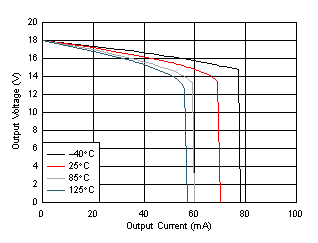Sourcing
Figure 6. Output Voltage Swing vs Output Current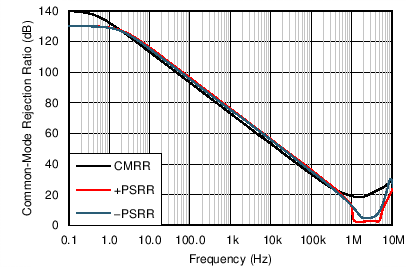Figure 8. CMRR and PSRR vs Frequency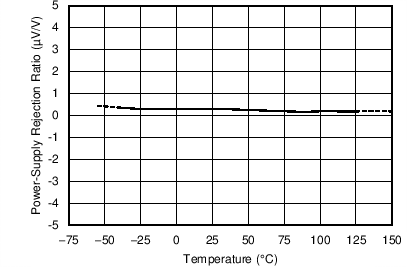Figure 10. PSRR vs Temperature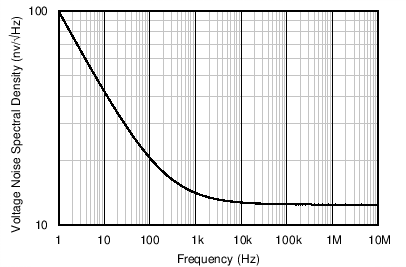Figure 12. Input Voltage Noise Spectral Density
vs Frequency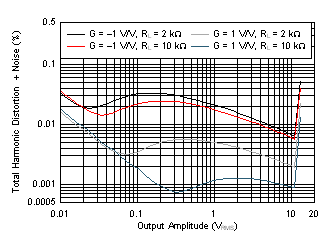Figure 14. THD+N vs Output AmplitudeFigure 16. Quiescent Current vs TemperatureRL = 2 kΩ
Figure 18. Open-Loop Gain vs Temperature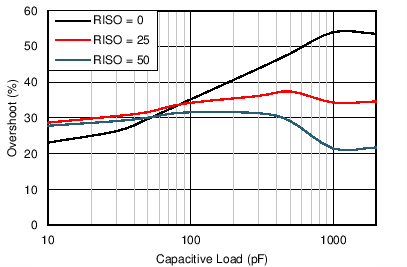G = –1, 100-mV output step
Figure 20. Small-Signal Overshoot vs Capacitive Load
(100-mV Output Step)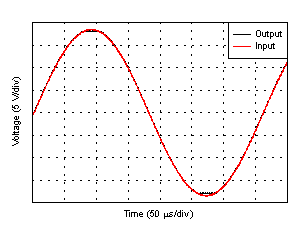Figure 22. No Phase Reversal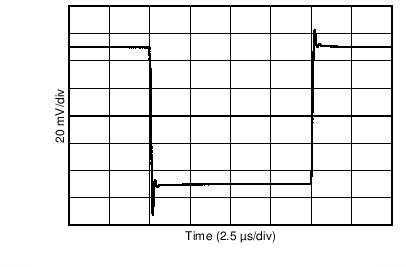G = 1, CL = 10 pF
Figure 24. Small-Signal Step ResponseG = 1, CL = 10 pF
Figure 26. Large-Signal Step Response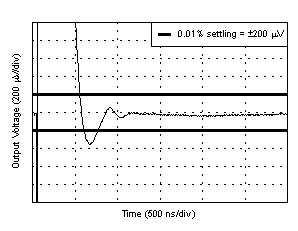Gain = 1, 2-V step, rising, step applied at t = 0 µs on all four plots
Figure 28. 0.01% Settling Time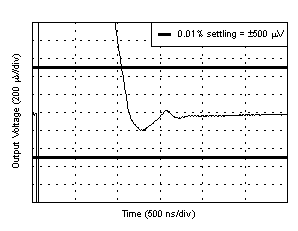Gain = 1, 5-V step, rising, step applied at t = 0 µs
Figure 30. 0.01% Settling Time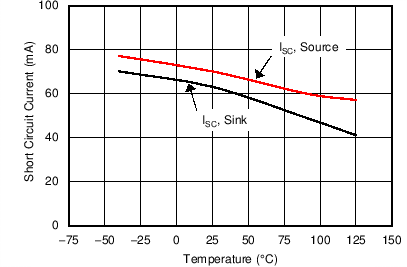Figure 32. Short-Circuit Current vs Temperature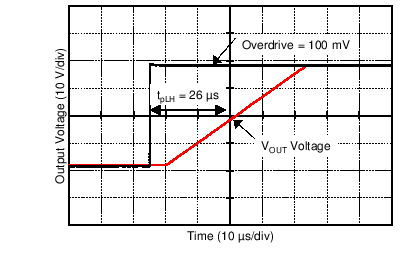Figure 34. Propagation Delay Rising Edge